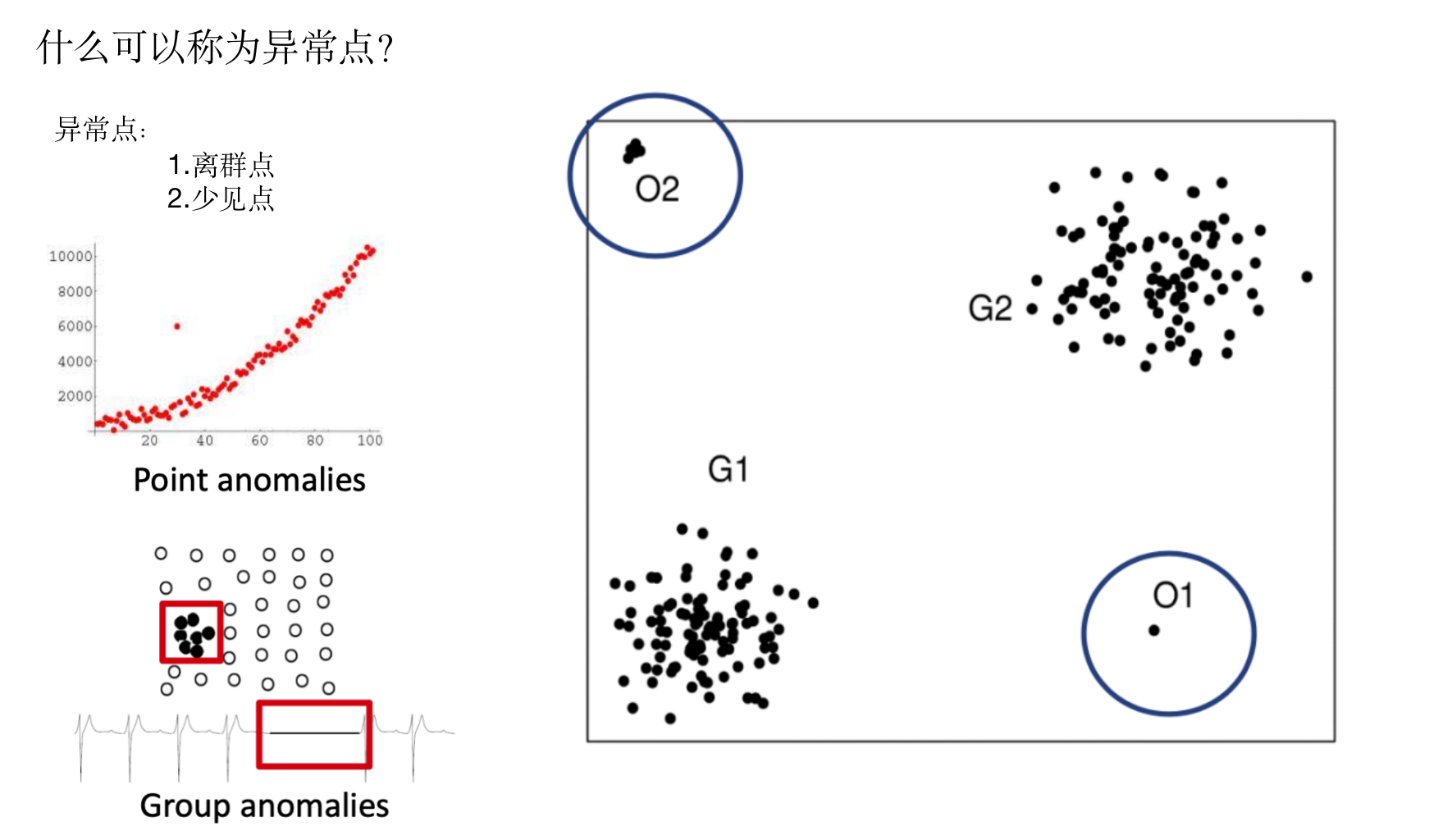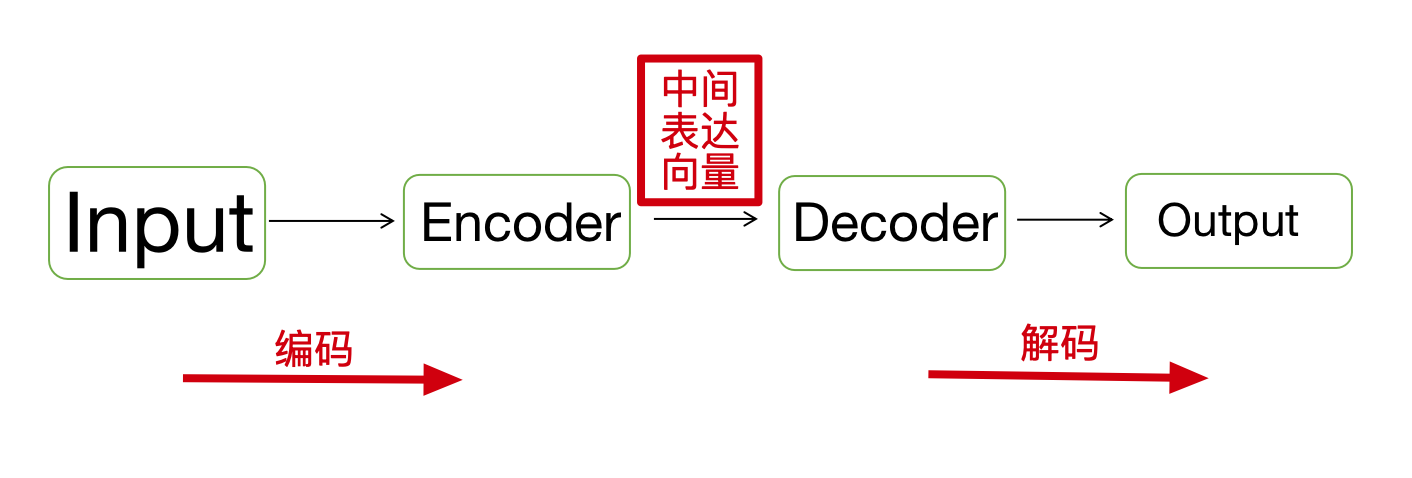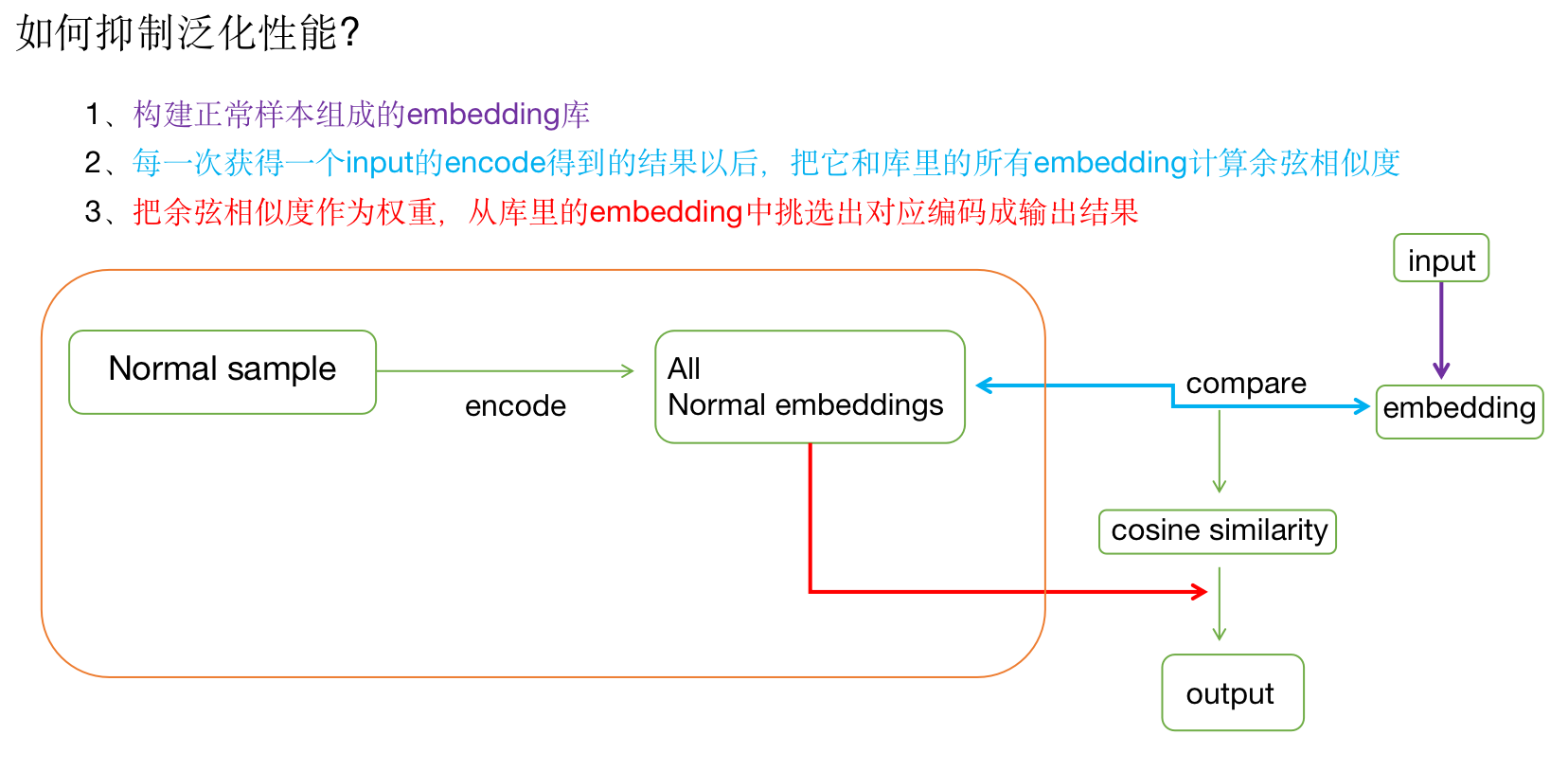# Anomaly Detection

@张一极

zk@likedge.top## 图像领域的异常样本：1、关于权重的计算：

paper中的embedding需要做一个转置，方便点积，$d\left(\mathbf{input_{emb}}, \mathbf{embedding}_{i}\right)=\frac{\mathbf{input_{emb}\cdot embedding}_{i}^{\top}}{\|\mathbf{input_{emb}}\|\left\|\mathbf{embedding}_{i}\right\|}$,输入embedding为$input_{emb}$$embedding_i$为库中第i个向量。

2、关于embedding个数的限制

$\widehat{w}_{i}=h\left(w_{i} ; \lambda\right)=\left\{\begin{array}{ll}w_{i}, & \text { if } w_{i}>\lambda \\ 0, & \text { otherwise }\end{array}\right.$，为使其可导，$\widehat{w}_{i}=\frac{\max \left(w_{i}-\lambda, 0\right) \cdot w_{i}}{\left|w_{i}-\lambda\right|+\epsilon}$

## GAN

1. 用generator重构图像，discriminator只是用来帮助generator更好地重构图像。在判断异常时，类似于auto-encoder检测异常的思路，用generator重构后的图像和原图做对比，不同处认为是异常；
2. 使用gan重建图像，在检测异常时，综合generator和discriminator的结果作为判断异常的依据。